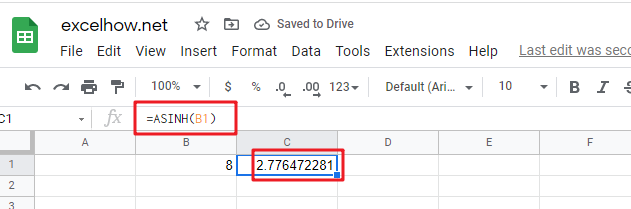This post will guide you how to use Google Sheets ASINH function with syntax and examples.

## Description

The Google Sheets ASINH function returns the inverse hyperbolic sine of a number.

The ASINH function is a build-in function in Google Sheets and it is categorized as a MATH function.

## Syntax

The syntax of the ASINH function is as below:

= ASINH (number)

Where the ASINH function argument is:

• Number – This is a required argument.  A numeric value

## Google Sheets ASINH Function Examples

The below examples will show you how to use google sheets ASINH function to return the arcsine value of a number.

#1 =ASINH(B1)